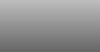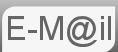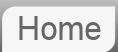# Answer to Puzzle #32: Two Creepers Climbing a Tree

32. Two creepers, one jasmine and other rose, are both climbing up and round a cylindrical tree trunk. Jasmine twists clockwise and rose anticlockwise, both start at the same point on the ground. Before they reach the first branch of the tree the jasmine had made 5 complete twists and the rose 3 twists. Not counting the bottom and the top, how many times do they cross?

This puzzle was emailed to me by Pramod S and solved by Pratik Poddar who runs a maths blog with many interesting puzzles that are slightly more mathematical than mine, his page on the same puzzle is here, there are a couple of different approaches there.

The first thing to realise is the vertical aspect of the puzzle is something of an illusion. For convenience we will proceed as if they both travel vertically at the same speed and thus complete the journey in the same unspecified time Ttotal, but this is for convenience regardless of the times the path described is the same.

From here we may now consider their motion as being constrained to the outer edge of a circle in the horizontal plane. The problem now becomes not dissimilar to a puzzle involving the overlap of the hands of a clock, though of course the hands are moving in opposite directions.

Each of the creepers will have speeds 5w and 3w. Where 'w' is some unspecified angular velocity in degrees per second, although the units are arbitrary. Using speed is distance over time or time = distance / speed, knowing that the total distance travelled 360 degrees times 5 revolutions or 3 revolutions we can easily show Ttotal as a function of w. Ttotal = 360 * 5 / (5w) or Ttotal = 360 * 3 / (3w) hence Ttotal = 360 / w. We will need this later.

## The First Coincidence

We now have two motions around a circle starting at one point, travelling in opposite directions, with speeds 3w and 5w. We need to solve this for time.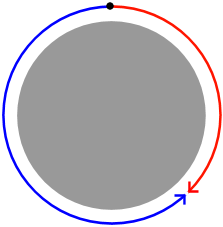We know a total distance of 360 degrees is travelled by two objects with speeds 5w and 3w. Our equations of motion give us:

360=5wt + 3wt = 8wt
t = 360 / 8w

So our first overlap is at t = 360 / 8w. In terms of subsequent overlaps the puzzle is in some ways reset. They are co-existent at this time and travelling in opposite directions. if we were to calculate the next time of overlap it would be the same. We now know an overlap occurs every 360 / 8w

## Total Coincidence

An overlap occurs every 360 / 8w for a time of Ttotal = 360 / w hence the number of coincidence is

:Ttotal / time between coincidence
:(360 / w) / (360 / 8w)
:8

since the division is exact we know that the last coincidence is at t = Ttotal, hence there are 7 overlaps not including the top and bottom.

## Simpler Model

Since they are moving in the opposite direction we can actually just add the two. 5 + 3 (minus the top and bottom.) This is a reference frame argument, for the justification we will use it again when we look at overlapping hands of a clock.

Bard first refused to answer. Then got it wrong.

If you're curious what Bard made of this puzzle...

If you're curious what ChatGPT made of this puzzle...

© Nigel Coldwell 2004 -  – The questions on this site may be reproduced without further permission, I do not claim copyright over them. The answers are mine and may not be reproduced without my expressed prior consent. Please inquire using the link at the top of the page. Secure version of this page.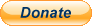PayPal
I always think it's arrogant to add a donate button, but it has been requested. If I help you get a job though, you could buy me a pint! - nigel